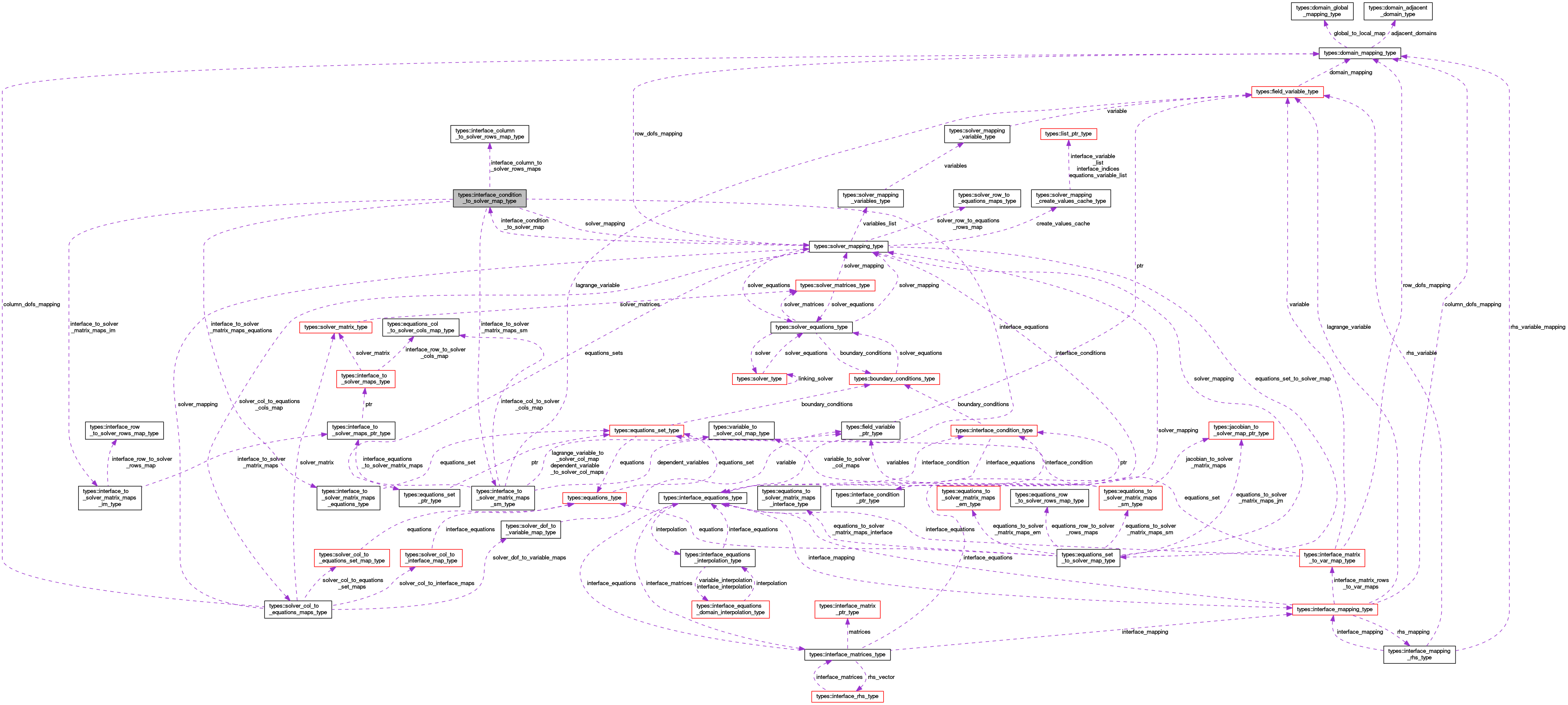OpenCMISS-Iron Internal API Documentation
types::interface_condition_to_solver_map_type Type Reference

Contains information on the mappings from an interface condition to the solver matrices. More...

Collaboration diagram for types::interface_condition_to_solver_map_type:[legend]

## Public Attributes

integer(intg) interface_condition_index
The index of the interface condition for these mappings. More...

type(solver_mapping_type), pointer solver_mapping
A pointer to the solver mappings. More...

type(interface_equations_type), pointer interface_equations
A pointer to the interface equations in this interface condition. More...

integer(intg) number_of_equations_sets
The number of equations sets that the interface condition affects. More...

type(interface_to_solver_matrix_maps_equations_type), dimension(:), allocatable interface_to_solver_matrix_maps_equations
INTERFACE_TO_SOLVER_MATRIX_MAPS_EQUATIONS(equations_set_idx). The equations set information of the equations_set_idx'th equations set that the interface condition affects. More...

type(interface_to_solver_matrix_maps_sm_type), dimension(:), allocatable interface_to_solver_matrix_maps_sm
INTERFACE_TO_SOLVER_MATRIX_MAPS_SM(solver_matrix_idx). The mappings from the interface matrices in this interface condition to the solver_matrix_idx'th solver_matrix. More...

type(interface_to_solver_matrix_maps_im_type), dimension(:), allocatable interface_to_solver_matrix_maps_im
INTERFACE_TO_SOLVER_MATRIX_MAPS_IM(interface_matrix_idx). The mappings from the interface_matrix_idx'th interface matrix in this interface condition to the solver_matrices. More...

type(interface_column_to_solver_rows_map_type), dimension(:), allocatable interface_column_to_solver_rows_maps
INTERFACE_COLUMN_TO_SOLVER_ROW_MAP(interface_column_idx). The mapping from the interface_column_idx'th interface column to a solver row. More...

## Detailed Description

Contains information on the mappings from an interface condition to the solver matrices.

Definition at line 2968 of file types.f90.

## Member Data Documentation

 type(interface_column_to_solver_rows_map_type), dimension(:), allocatable types::interface_condition_to_solver_map_type::interface_column_to_solver_rows_maps

INTERFACE_COLUMN_TO_SOLVER_ROW_MAP(interface_column_idx). The mapping from the interface_column_idx'th interface column to a solver row.

Definition at line 2976 of file types.f90.

 integer(intg) types::interface_condition_to_solver_map_type::interface_condition_index

The index of the interface condition for these mappings.

Definition at line 2969 of file types.f90.

 type(interface_equations_type), pointer types::interface_condition_to_solver_map_type::interface_equations

A pointer to the interface equations in this interface condition.

Definition at line 2971 of file types.f90.

 type(interface_to_solver_matrix_maps_equations_type), dimension(:), allocatable types::interface_condition_to_solver_map_type::interface_to_solver_matrix_maps_equations

INTERFACE_TO_SOLVER_MATRIX_MAPS_EQUATIONS(equations_set_idx). The equations set information of the equations_set_idx'th equations set that the interface condition affects.

Definition at line 2973 of file types.f90.

 type(interface_to_solver_matrix_maps_im_type), dimension(:), allocatable types::interface_condition_to_solver_map_type::interface_to_solver_matrix_maps_im

INTERFACE_TO_SOLVER_MATRIX_MAPS_IM(interface_matrix_idx). The mappings from the interface_matrix_idx'th interface matrix in this interface condition to the solver_matrices.

Definition at line 2975 of file types.f90.

 type(interface_to_solver_matrix_maps_sm_type), dimension(:), allocatable types::interface_condition_to_solver_map_type::interface_to_solver_matrix_maps_sm

INTERFACE_TO_SOLVER_MATRIX_MAPS_SM(solver_matrix_idx). The mappings from the interface matrices in this interface condition to the solver_matrix_idx'th solver_matrix.

Definition at line 2974 of file types.f90.

 integer(intg) types::interface_condition_to_solver_map_type::number_of_equations_sets

The number of equations sets that the interface condition affects.

Definition at line 2972 of file types.f90.

 type(solver_mapping_type), pointer types::interface_condition_to_solver_map_type::solver_mapping

A pointer to the solver mappings.

Definition at line 2970 of file types.f90.